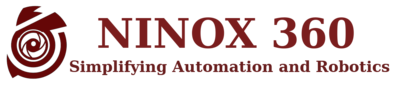# Main Page

 BoofCV is an open source library written from scratch for real-time computer vision. Its functionality covers a range of subjects, low-level image processing, camera calibration, feature detection/tracking, structure-from-motion, fiducial detection, and recognition. BoofCV has been released under an Apache 2.0 license for both academic and commercial use. BoofCV is organized into several packages: image processing, features, geometric vision, calibration, recognition,visualize, and IO. Image processing contains commonly used image processing functions which operate directly on pixels. Features contains feature extraction algorithms for use in higher level operations. Calibration has routines for determining the camera's intrinsic and extrinsic parameters. Recognition is for recognition and tracking complex visual objects. Geometric vision is composed of routines for processing extracted image features using 2D and 3D geometry. Visualize has routines for rendering and displaying extracted features. IO has input and output routines for different data structures.A start up from the creators of BoofCV.

Prebuild Stand Alone Applications

 Examples / Demonstrations Example and demonstration applications QR Code Generator Create and print QR Codes Square Fiducials Binary/Image Square Fiducial Generator Calibration Targets Calibration Target Generator Calibration Camera Calibration Application Lens Undistortion Batch Removal of Lens Distortion Image Downsize Batch Image Down Sampling with minimal aliasing

## Capability Summary

Image Processing Feature Recognition Geometric Integration
 Image Convolution Example Image Derivatives Example Thresholding Example Binary Ops Example Color Space Example Interpolation Example Image Deformation Example Image Blur Example Enhancement Example Noise Removal Example Fourier Transform Example Wavelet Decomposition Discrete Image Pyramid Example Float Image Pyramid Example Scale Space Image Equirectangular Example Fisheye Cameras Example
 Black Polygons Example Black Ellipses Example Interest Points Example Line Detection Example Motion Detection Example Binary Contours Example Polygon Fitting Example Ellipse Fitting Example Template Matching Example Interest Points Example Dense Features Example Point Tracking Example Non-max Suppression Example Dense Optical Flow Example Superpixels Example Color Segmentation Example
 Scene Recognition Example Color Image Lookup Example KNN Classifier Example CNN Classifiers Example Object Tracking Example Fiducials Tutorial QR Codes Tutorial
 SFM Tutorial Camera Calibration Tutorial Mono Calibration Example Fisheye Calibration Example Stereo Calibration Example Remove Distortion Example Equi to Pinhole Example Fisheye to Equi Example Bundle Adjustment Example 3D Stereo Cloud Example Monocular Stereo Example Uncalibrated Stereo Example Multi Baseline Stereo Example Scene Reconstruction Example Video Stabilization Example Video Mosaic Example Visual Odom: Stereo Example Visual Odom: Mono Plane Example Visual Odom: RGB-D Example
 Visualization Android Video Kinect Processing Python